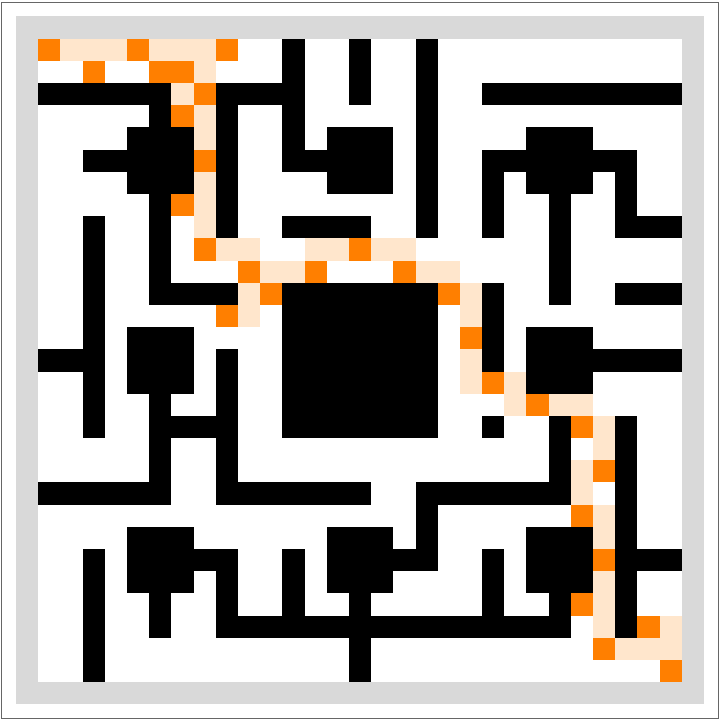Function Repository Resource:

# RandomSierpinskiMaze

Generate a maze based on the Sierpinski carpet

 ResourceFunction["RandomSierpinskiMaze"][scale] returns the ArrayPlot of a maze drawn by connecting dots on a square fragment of the Sierpiński carpet fractal, whose length dimension roughly equals 3scale+1. ResourceFunction["RandomSierpinskiMaze"][scale, AdjacencyGraph] returns a primitive Graph of the maze, which determines allowable moves on the corresponding ArrayPlot.

## Details

The image dimension is equal to {4+3scale+1,4+3scale+1} due to extra padding around the edges.

## Examples

### Basic Examples (3)

Depict a basic unit of the randomized maze:

 In:=Out=Scale up the basic unit by areal factors of 9:

 In:=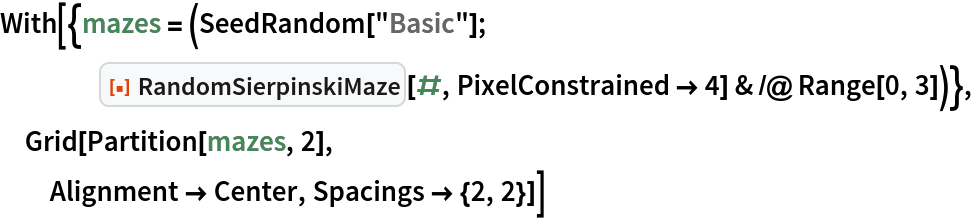Out=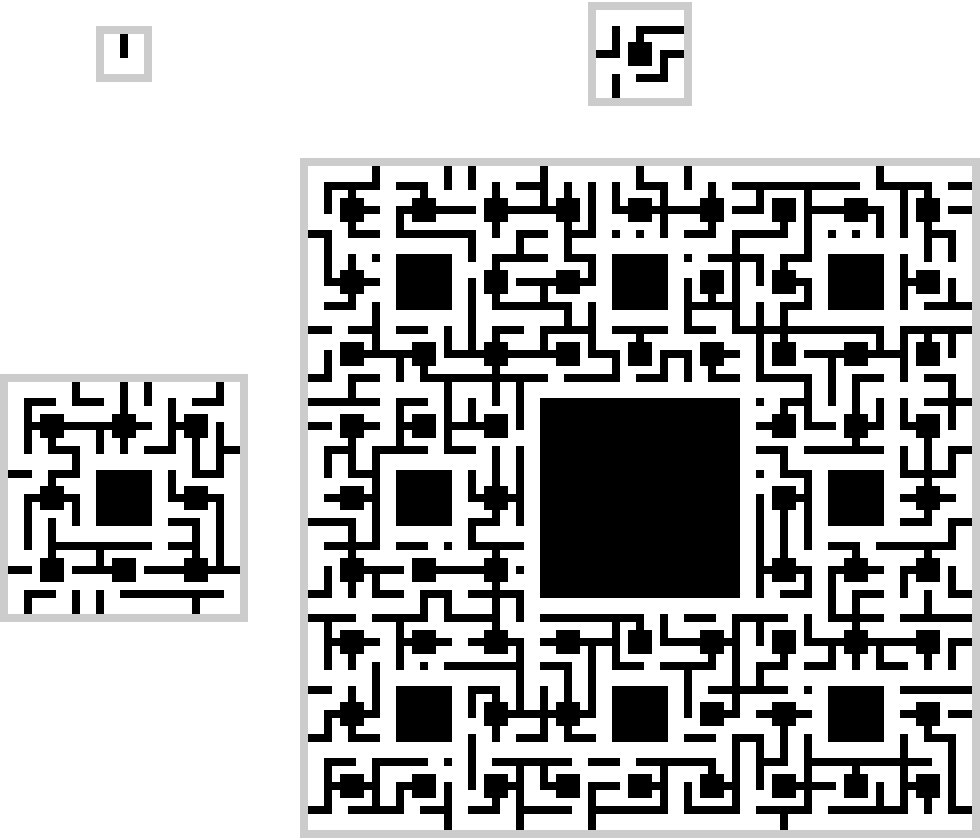Compare the ArrayPlot with its primitive Graph:

 In:=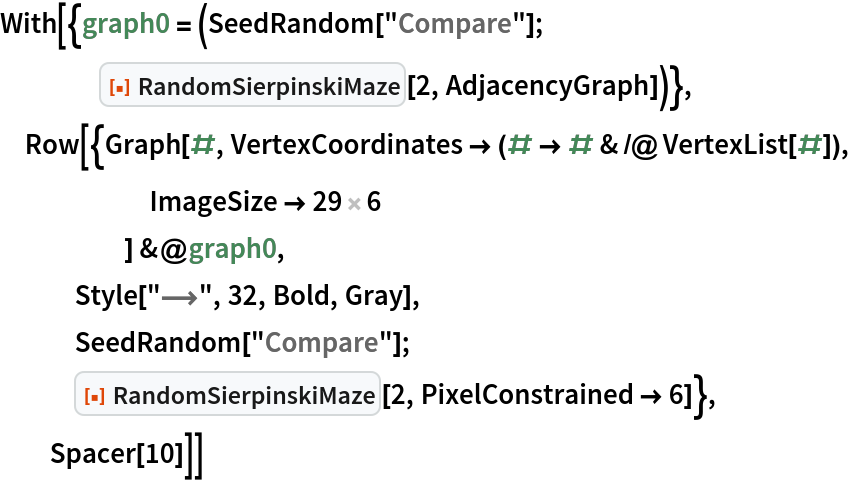Out=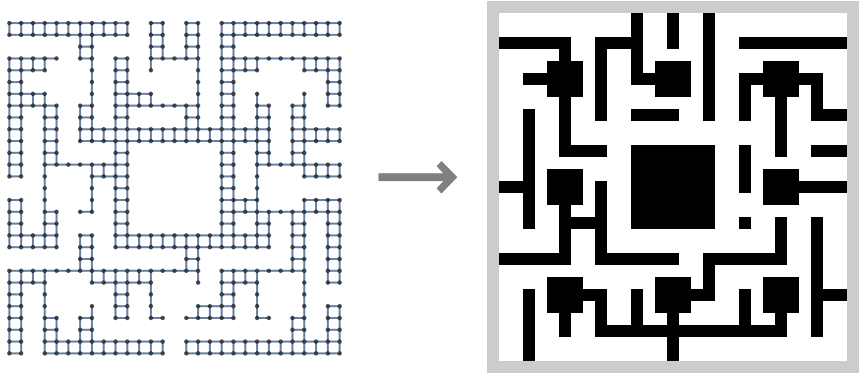Display the same Graph coordinate-free and highlight a shortest path between corners:

 In:=Out=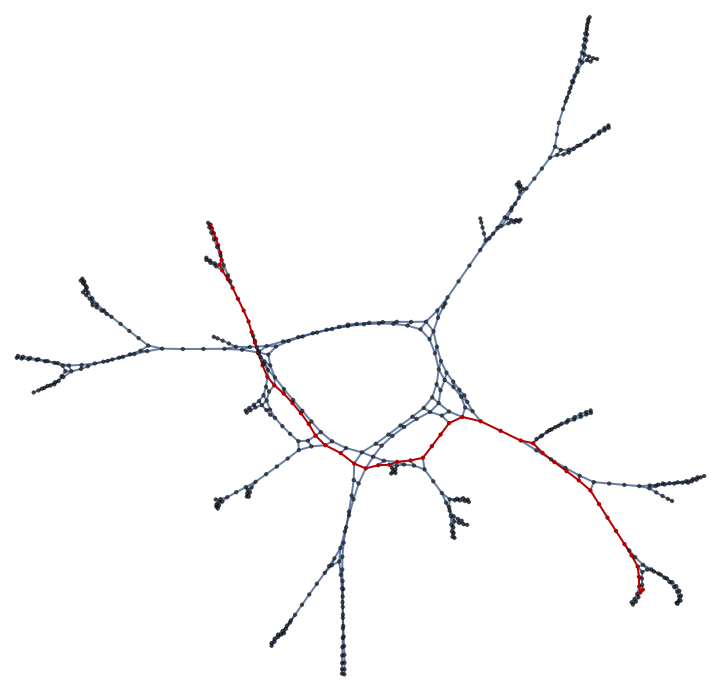Depict the path in an ArrayPlot:

 In:=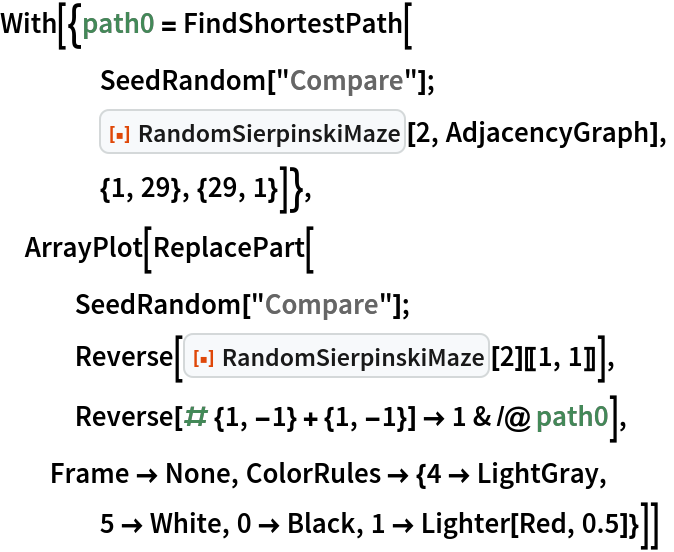Out=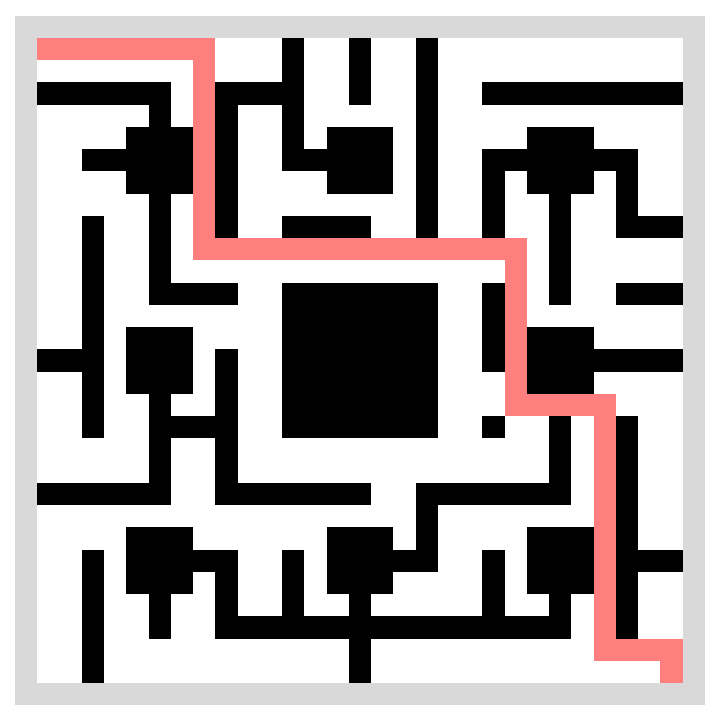### Scope (1)

The measured complexity is super-exponential with regard to input scale, but it is possible to obtain mazes up to scale=4 in reasonable time:

 In:=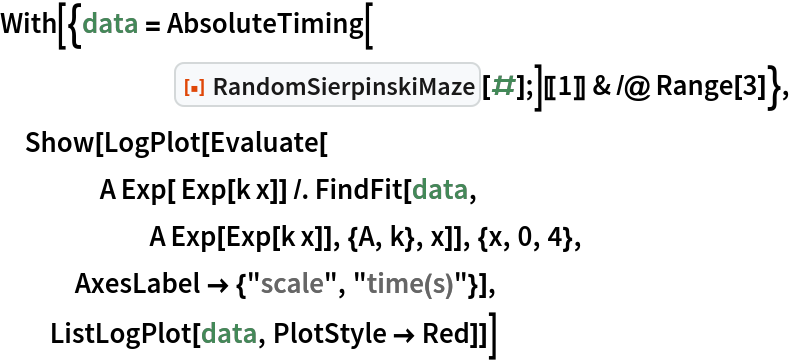Out=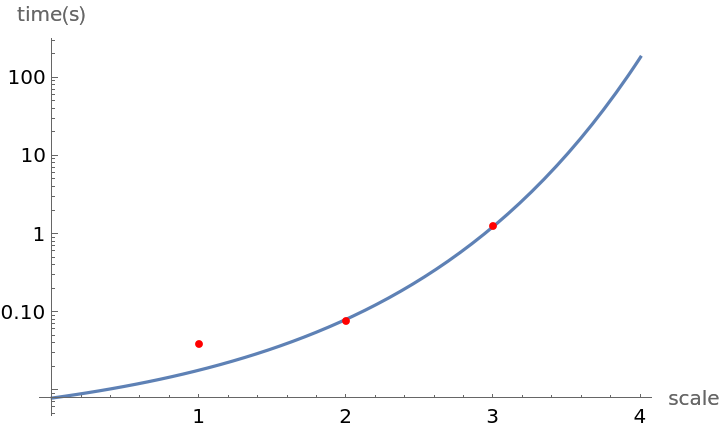### Neat Examples (2)

Use ArrayMesh to build a maze out of voxels:

 In:=In:=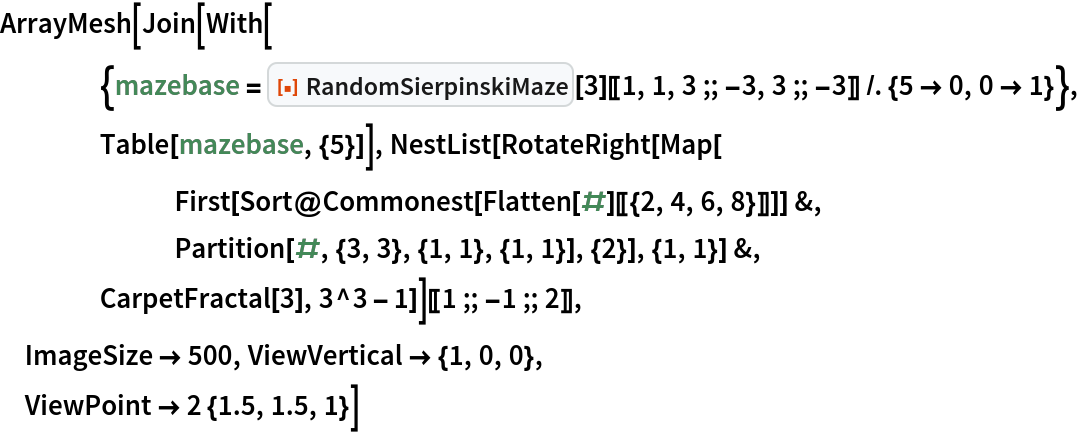Out=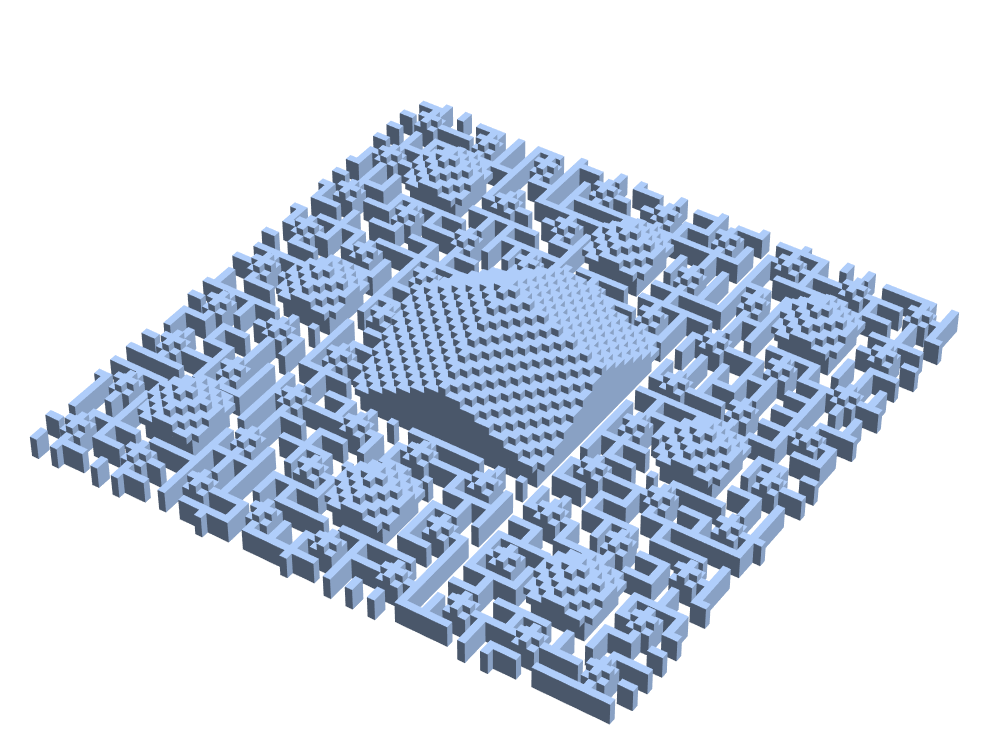Transform output Graph to a knight's walk graph:

 In:=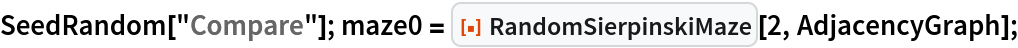In:=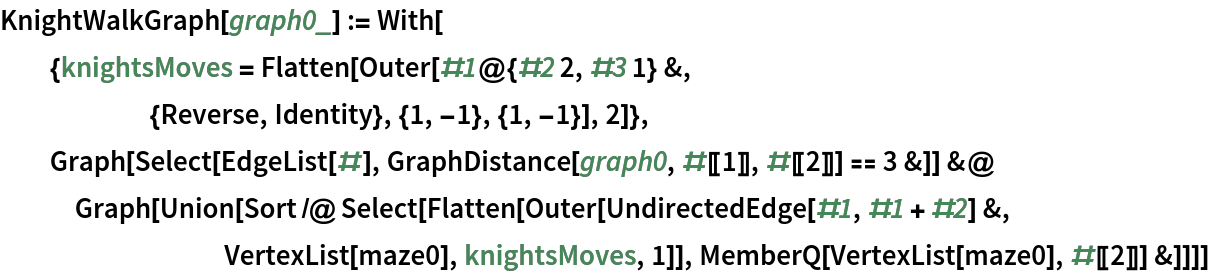In:=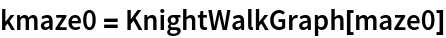Out=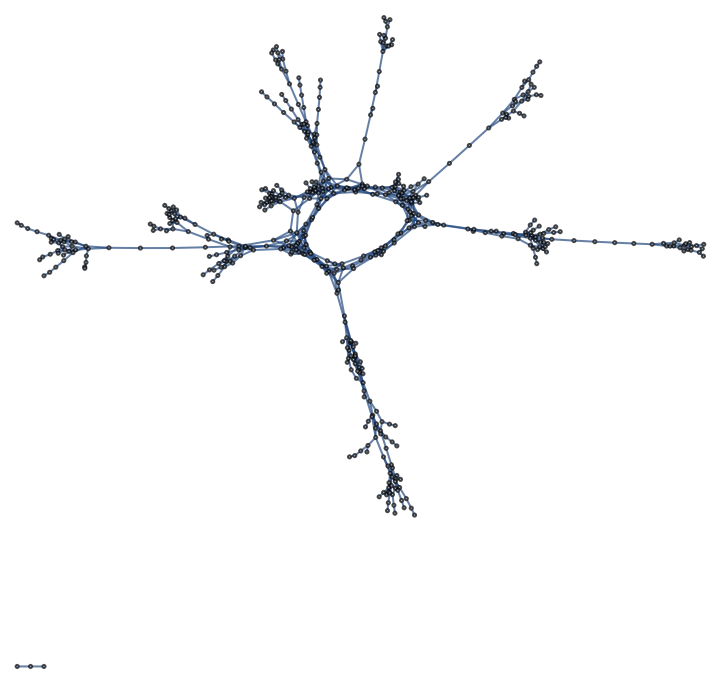Draw the knight's walk Graph over the maze adjacency Graph:

 In:=Out=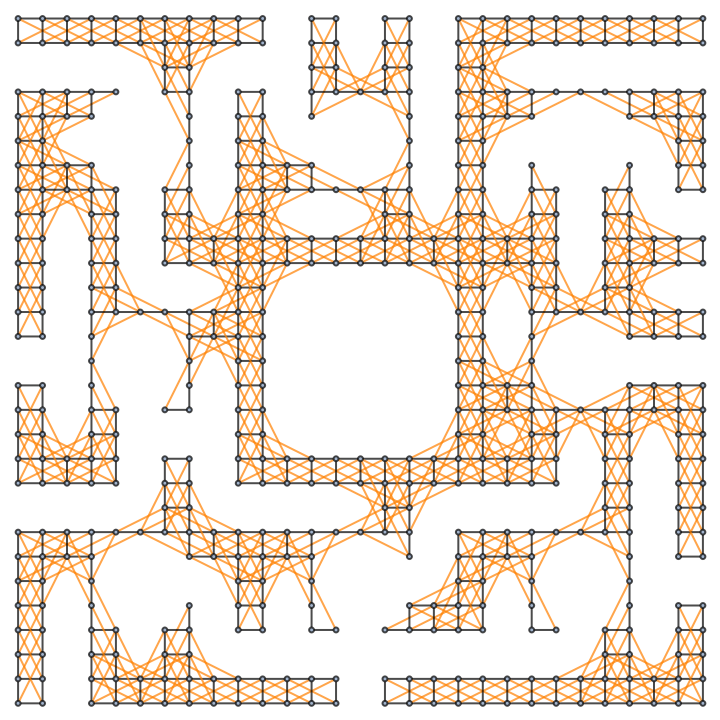Depict a knight's shortest path between corners:

 In:=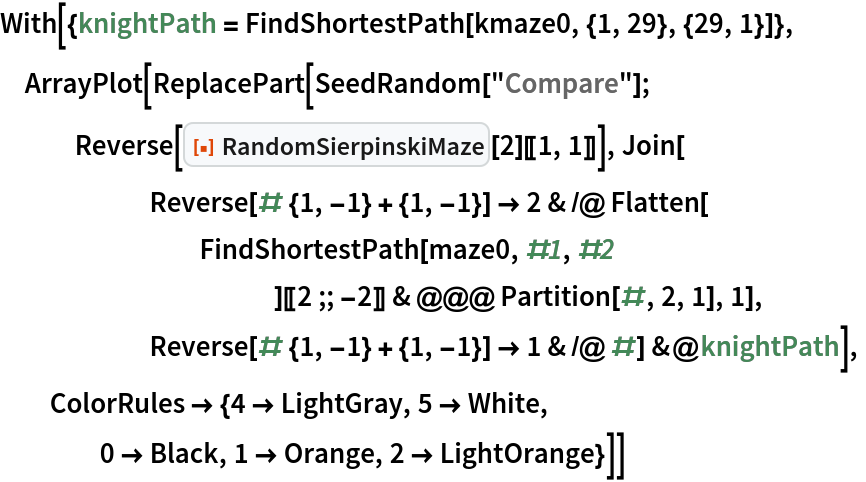Out=